# Q4: Solve Bernoulli's equation dy + 3xy = y2 x3 : dx (10 Marks)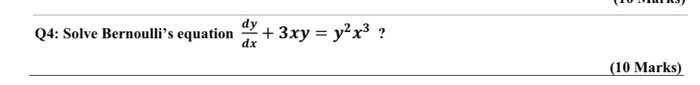Q4: Solve Bernoulli's equation dy + 3xy = y2 x3 : dx (10 Marks)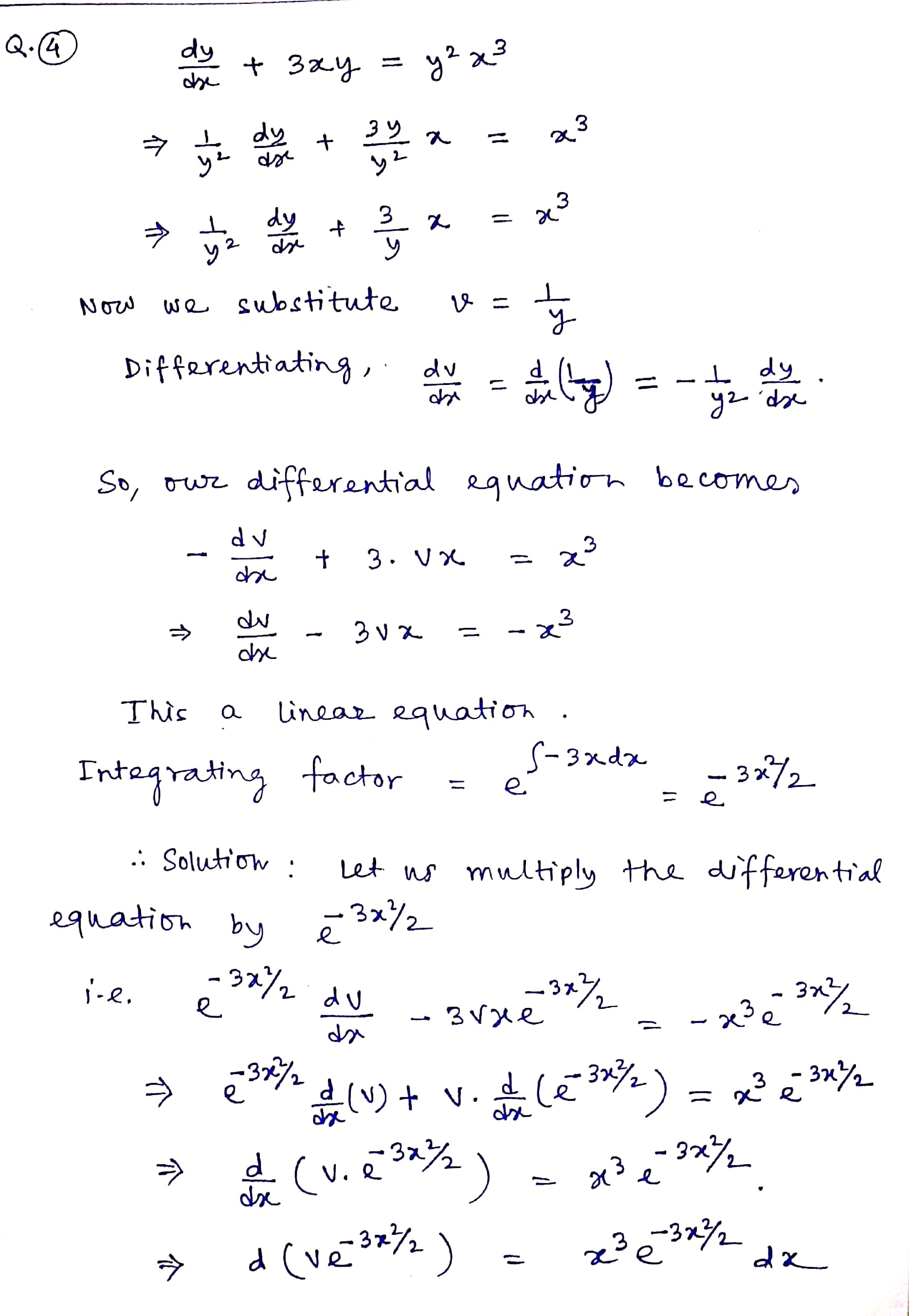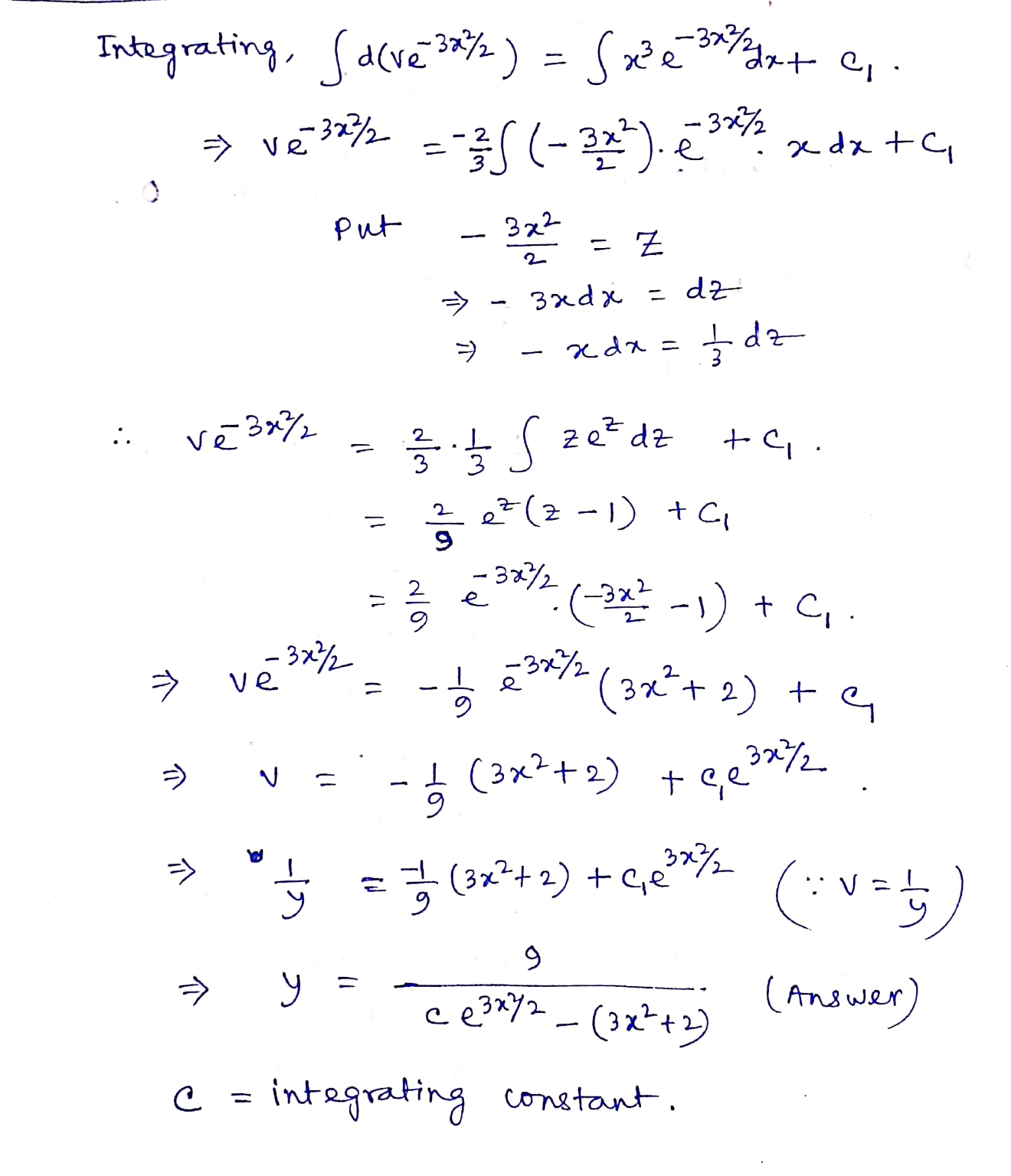##### Add Answer of: Q4: Solve Bernoulli's equation dy + 3xy = y2 x3 : dx (10 Marks)
Similar Homework Help Questions
• ### Q4: Solve Bernoulli's equation dy + 3xy = y2x3 : dx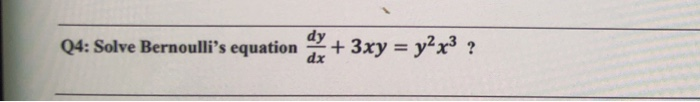Q4: Solve Bernoulli's equation dy + 3xy = y2x3 : dx

• ### Q4: Solve Bernoulli's equation dy + 3xy = y2x3 ? dx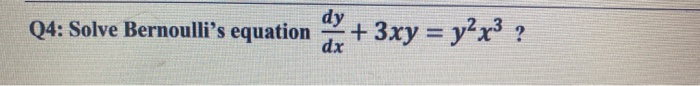Q4: Solve Bernoulli's equation dy + 3xy = y2x3 ? dx

• ### the Q is in the photo Q4: Solve Bernoulli's equation dy dx + 3xy = y2x3...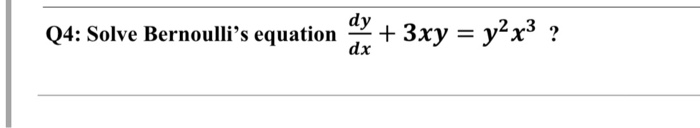the Q is in the photo Q4: Solve Bernoulli's equation dy dx + 3xy = y2x3 ?

• ### dy 04: Solve Bernoulli's equation dx + 3xy = y2x32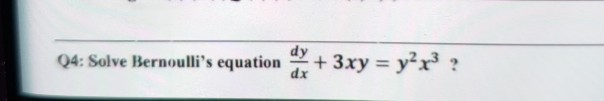dy 04: Solve Bernoulli's equation dx + 3xy = y2x32

• ### Q3/ Evaluate the following double integral by using Simpson's Rule Method 2 2 3xy) etx dx dy (x3 -2 0 Number interv...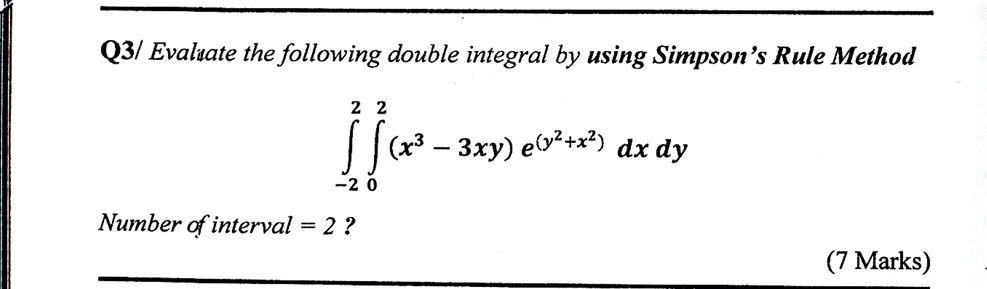Q3/ Evaluate the following double integral by using Simpson's Rule Method 2 2 3xy) etx dx dy (x3 -2 0 Number interval 2 ? of (7 Marks) Q3/ Evaluate the following double integral by using Simpson's Rule Method 2 2 3xy) etx dx dy (x3 -2 0 Number interval 2 ? of (7 Marks)

• ### Find the G.S. of the DE: (3xy - y2)dx + x(x - y)dy = 0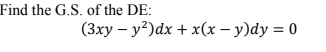Find the G.S. of the DE: (3xy - y2)dx + x(x - y)dy = 0

• ### Solve the separable differential equation (y2+2)dx+y(x+4)dy=0.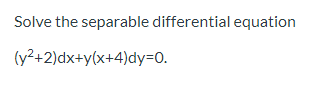Solve the separable differential equation (y2+2)dx+y(x+4)dy=0.

• ### Differential Equations: Bernoulli's Equation

2(dy/dx) + y = y3(x-1)Use Bernoulli's Equation to solve as far as possible

• ### Solve the differential equation. dy dx 3.c2e- y=In(x3 + C) y=Cln(x) y=+*+ Oy=ln(3x + C)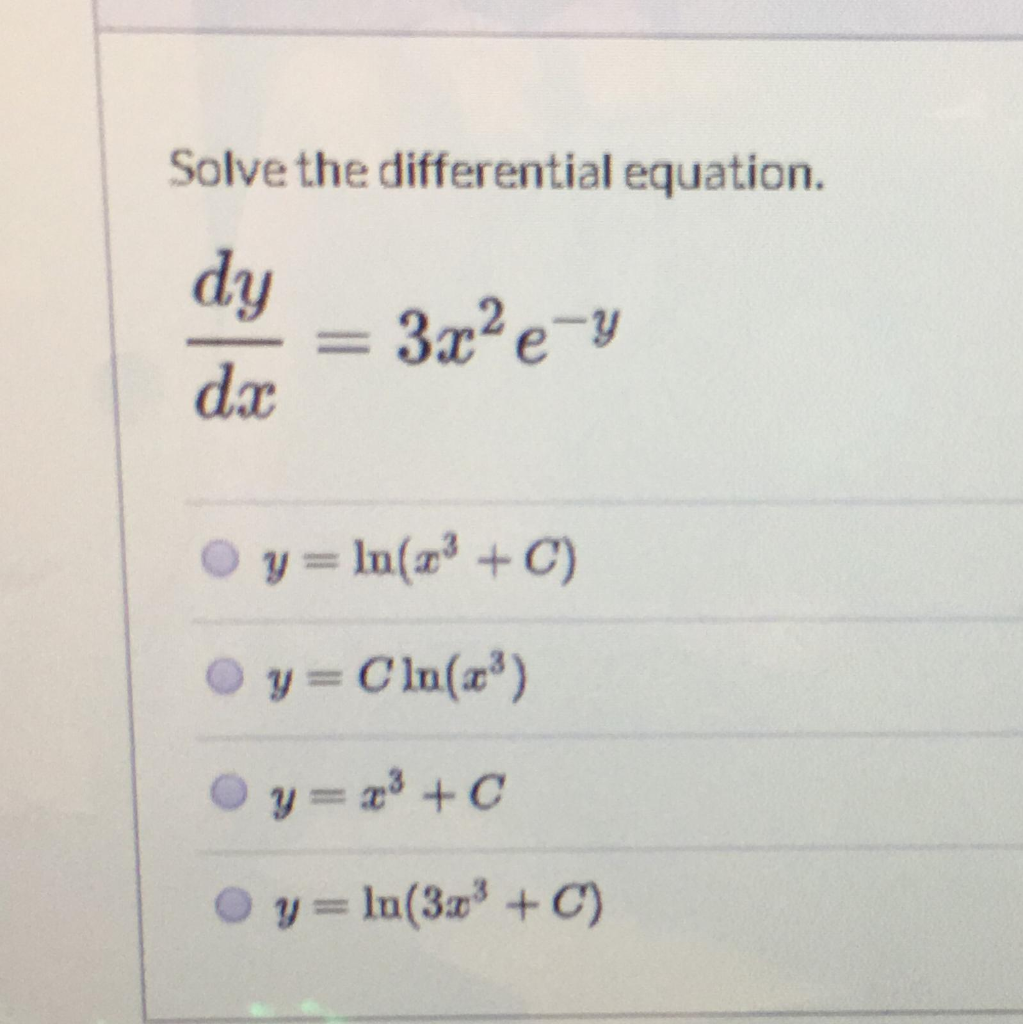Solve the differential equation. dy dx 3.c2e- y=In(x3 + C) y=Cln(x) y=+*+ Oy=ln(3x + C)

• ### Q4/ Solve the following ordinary differential equation: (ex+y + y ey) dx + (xey - 1...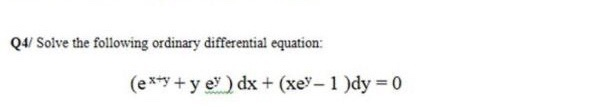Q4/ Solve the following ordinary differential equation: (ex+y + y ey) dx + (xey - 1 )dy = 0

Free Homework App